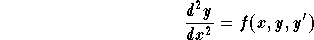#Second Order Differential EquationsA second order differential equation is an equation involving the unknown function y, its derivatives y' and y'', and the variable x. We will only consider explicit differential equations of the form,[Differential Equations]
[Geometry] [Algebra] [Trigonometry ]
[Calculus] [Complex Variables] [Matrix Algebra]S.O.S MATHematics home page

Do you need more help? Please post your question on our S.O.S. Mathematics CyberBoard.Author: Mohamed Amine Khamsi